Dan Crisan

Professor of Mathematics

Imperial College London

Department of Mathematics

180 Queen's Gate

London SW7 2AZ, UK

d.crisan@imperial.ac.uk

0207 594 8489

Stochastic Analysis Group

Books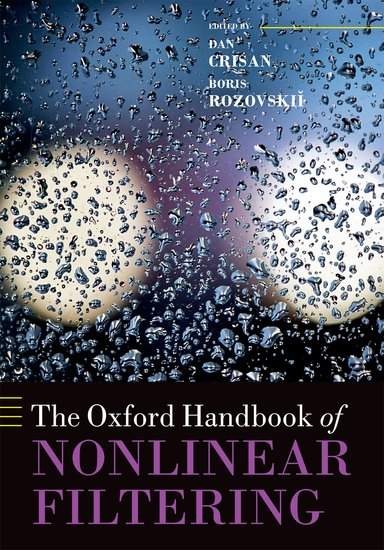The Oxford Handbook of Nonlinear FilteringMathematics of Planet Earth A Primer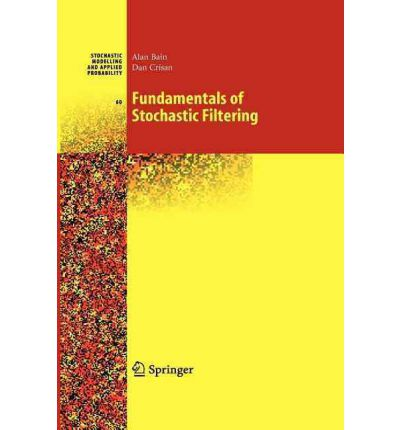Fundamentals of Stochastic Filtering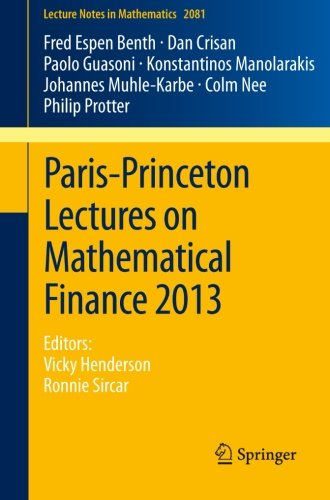Paris-Princeton Lectureson MathematicalFinance 2013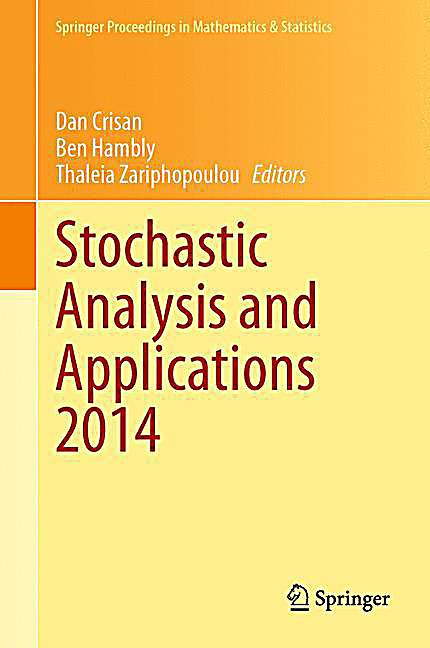Stochastic Analysis and applications 2014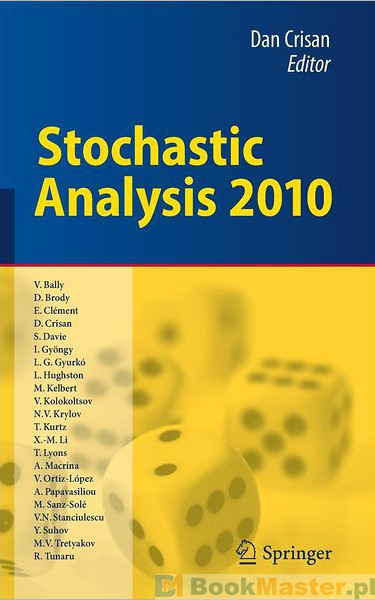Description of the books:

The Oxford Handbook of Nonlinear Filtering

In many areas of human endeavour, the systems involved are not available for direct measurement. Instead, by combining mathematical models for a system's evolution with partial observations of its evolving state, we can make reasonable inferences about it. The increasing complexity of the modern world makes this analysis and synthesis of high-volume data an essential feature in many real-world problems. The celebrated Kalman-Bucy filter, designed for linear dynamical systems with linearly structured measurements, is the most famous Bayesian filter. Its generalizations to nonlinear systems and/or observations are collectively referred to as nonlinear filtering (NLF), an extension of the Bayesian framework to the estimation, prediction, and interpolation of nonlinear stochastic dynamics. NLF uses a stochastic model to make inferences about an evolving system and is a theoretically optimal algorithm. The breadth of its applications, firmly established and still emerging, is simply astounding. Early uses such as cryptography, tracking, and guidance were mostly of a military nature. Since then, the scope has exploded. It includes the study of global climate, estimating the state of the economy, identifying tumours using non-invasive methods, and much more.

The Oxford Handbook of Nonlinear Filtering is the first comprehensive written resource for the subject. It contains classical and recent results and applications, with contributions from 58 authors. Collated into 10 parts, it covers the foundations of nonlinear filtering, connections to stochastic partial differential equations, stability and asymptotic analysis, estimation and control, approximation theory and numerical methods for solving the nonlinear filtering problem (including particle methods). It also contains a part dedicated to the application of nonlinear filtering to several problems in mathematical finance.

Mathematics of Planet Earth. A Primer

Mathematics of Planet Earth (MPE) started and continues to be consolidated as a collaboration of mathematical science organisations around the world. These organisations work together to tackle global environmental, social and economic problems using mathematics. This textbook introduces the fundamental topics of MPE to advanced undergraduate and graduate students in mathematics, physics and engineering while explaining their modern usages and operational connections. In particular, it discusses the links between partial differential equations, data assimilation, dynamical systems, mathematical modelling and numerical simulations and applies them to insightful examples.

The text also complements advanced courses in geophysical fluid dynamics (GFD) for meteorology, atmospheric science and oceanography. It links the fundamental scientific topics of GFD with their potential usage in applications of climate change and weather variability. The immediacy of examples provides an excellent introduction for experienced researchers interested in learning the scope and primary concepts of MPE.

Fundamentals of Stochastic Filtering

The objective of stochastic filtering is to determine the best estimate for the state of a stochastic dynamical system from partial observations. The solution of this problem in the linear case is the well known Kalman-Bucy filter which has found widespread practical application. The purpose of this book is to provide a rigorous mathematical treatment of the non-linear stochastic filtering problem using modern methods. Particular emphasis is placed on the theoretical analysis of numerical methods for the solution of the filtering problem via particle methods.

The book should provide sufficient background to enable study of the recent literature. While no prior knowledge of stochastic filtering is required, readers are assumed to be familiar with measure theory, probability theory and the basics of stochastic processes. Most of the technical results that are required are stated and proved in the appendices. The book is intended as a reference for graduate students and researchers interested in the field. It is also suitable for use as a text for a graduate level course on stochastic filtering. Suitable exercises and solutions are included.

Paris-Princeton Lectures on Mathematical Finance 2013

The current volume presents four chapters touching on some of the most important and modern areas of research in Mathematical Finance: asset price bubbles (by Philip Protter); energy markets (by Fred Espen Benth); investment under transaction costs (by Paolo Guasoni and Johannes Muhle-Karbe); and numerical methods for solving stochastic equations (by Dan Crisan, K. Manolarakis and C. Nee).The Paris-Princeton Lecture Notes on Mathematical Finance, of which this is the fifth volume, publish cutting-edge research in self-contained, expository articles from renowned specialists. The aim is to produce a series of articles that can serve as an introductory reference source for research in the field.

Stochastic Analysis and Applications 2014

Stochastic Analysis is the branch of mathematics that deals with the analysis of dynamical systems affected by noise. It emerged as a core area of mathematics in the late 20th century and has subsequently developed into an important theory with a wide range of powerful and novel tools, and with impressive applications within and beyond mathematics. Many systems are profoundly affected by stochastic fluctuations and it is not surprising that the array of applications of Stochastic Analysis is vast and touches on many aspects of life.

Articles from many of the main contributors to recent progress in stochastic analysis are included in this volume, which provides a snapshot of the current state of the area and its ongoing developments. It constitutes the proceedings of the conference on "Stochastic Analysis and Applications" held at the University of Oxford and the Oxford-Man Institute during 23-27 September, 2013.

The present volume is intended for researchers and Ph.D. students in stochastic analysis and its applications, stochastic optimization and financial mathematics, as well as financial engineers and quantitative analysts.

Stochastic Analysis 2010

Stochastic Analysis aims to provide mathematical tools to describe and model high dimensional random systems. Such tools arise in the study of Stochastic Differential Equations and Stochastic Partial Differential Equations, Infinite Dimensional Stochastic Geometry, Random Media and Interacting Particle Systems, Super-processes, Stochastic Filtering, Mathematical Finance, etc. Stochastic Analysis has emerged as a core area of late 20th century Mathematics and is currently undergoing a rapid scientific development.

The special volume "Stochastic Analysis 2010" provides a sample of the current research in the different branches of the subject. It includes the collected works of the participants at the Stochastic Analysis section of the 7th ISAAC Congress organized at Imperial College London in July 2009.